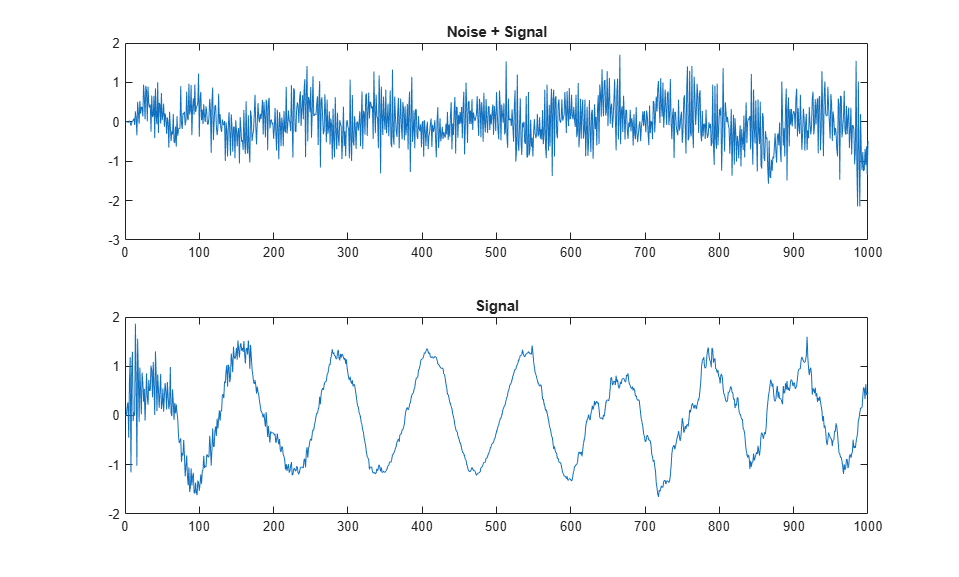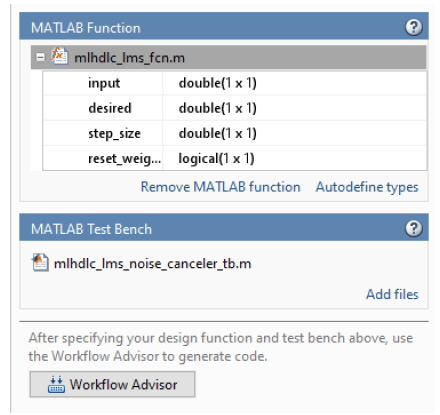# HDL Code Generation for LMS Filter

This example shows how to generate HDL code from a MATLAB® design that implements an LMS filter. The example also illustrates how to design a test bench that cancels out the noise signal by using this filter.

### LMS Filter MATLAB Design

The MATLAB design used in the example is an implementation of an LMS (Least Mean Squares) filter. The LMS filter is a class of adaptive filter that identifies an FIR filter signal that is embedded in the noise. The LMS filter design implementation in MATLAB consists of a top-level function `mlhdlc_lms_fcn` that calculates the optimal filter coefficients to reduce the difference between the output signal and the desired signal.

```design_name = 'mlhdlc_lms_fcn'; testbench_name = 'mlhdlc_lms_noise_canceler_tb'; ```

Review the MATLAB design:

```open(design_name); ```
```%%%%%%%%%%%%%%%%%%%%%%%%%%%%%%%%%%%%%%%%%%%%%%%%%%%%%%%%%%%%%%%%%%%%%%%%%% % MATLAB Design: Adaptive Noise Canceler algorithm using Least Mean Square % (LMS) filter implemented in MATLAB % % Key Design pattern covered in this example: % (1) Use of function calls % (2) Function inlining vs instantiation knobs available in the coder % (3) Use of system objects in the testbench to stream test vectors into the design %%%%%%%%%%%%%%%%%%%%%%%%%%%%%%%%%%%%%%%%%%%%%%%%%%%%%%%%%%%%%%%%%%%%%%%%%% %#codegen function [filtered_signal, y, fc] = mlhdlc_lms_fcn(input, ... desired, step_size, reset_weights) % 'input' : The signal from Exterior Mic which records the ambient noise. % 'desired': The signal from Pilot's Mic which includes % original music signal and the noise signal % 'err_sig': The difference between the 'desired' and the filtered 'input' % It represents the estimated music signal (output of this block) % % The LMS filter is trying to retrieve the original music signal('err_sig') % from Pilot's Mic by filtering the Exterior Mic's signal and using it to % cancel the noise in Pilot's Mic. The coefficients/weights of the filter % are updated(adapted) in real-time based on 'input' and 'err_sig'. % register filter coefficients persistent filter_coeff; if isempty(filter_coeff) filter_coeff = zeros(1, 40); end % Variable Filter: Call 'tapped_delay_fcn' function on path to create % 40-step tapped delay delayed_signal = mtapped_delay_fcn(input); % Apply filter coefficients weight_applied = delayed_signal .* filter_coeff; % Call treesum function on matlab path to sum up the results filtered_signal = mtreesum_fcn(weight_applied); % Output estimated Original Signal td = desired; tf = filtered_signal; esig = td - tf; y = esig; % Update Weights: Call 'update_weight_fcn' function on MATLAB path to % calculate the new weights updated_weight = update_weight_fcn(step_size, esig, delayed_signal, ... filter_coeff, reset_weights); % update filter coefficients register filter_coeff = updated_weight; fc = filter_coeff; function y = mtreesum_fcn(u) %Implement the 'sum' function without a for-loop % y = sum(u); % The loop based implementation of 'sum' function is not ideal for % HDL generation and results in a longer critical path. % A tree is more efficient as it results in % delay of log2(N) instead of a delay of N delay % This implementation shows how to explicitly implement the vector sum in % a tree shape to enable hardware optimizations. % The ideal way to code this generically for any length of 'u' is to use % recursion but it is not currently supported by MATLAB Coder % NOTE: To instruct MATLAB Coder to compile an external function, % add the following compilation directive or pragma to the function code %#codegen % This implementation is hardwired for a 40tap filter. level1 = vsum(u); level2 = vsum(level1); level3 = vsum(level2); level4 = vsum(level3); level5 = vsum(level4); level6 = vsum(level5); y = level6; function output = vsum(input) coder.inline('always'); vt = input(1:2:end); for i = int32(1:numel(input)/2) k = int32(i*2); vt(i) = vt(i) + input(k); end output = vt; function tap_delay = mtapped_delay_fcn(input) % The Tapped Delay function delays its input by the specified number % of sample periods, and outputs all the delayed versions in a vector % form. The output includes current input % NOTE: To instruct MATLAB Coder to compile an external function, % add the following compilation directive or pragma to the function code %#codegen persistent u_d; if isempty(u_d) u_d = zeros(1,40); end u_d = [u_d(2:40), input]; tap_delay = u_d; function weights = update_weight_fcn(step_size, err_sig, ... delayed_signal, filter_coeff, reset_weights) % This function updates the adaptive filter weights based on LMS algorithm % Copyright 2007-2022 The MathWorks, Inc. % NOTE: To instruct MATLAB Coder to compile an external function, % add the following compilation directive or pragma to the function code %#codegen step_sig = step_size .* err_sig; correction_factor = delayed_signal .* step_sig; updated_weight = correction_factor + filter_coeff; if reset_weights weights = zeros(1,40); else weights = updated_weight; end ```

The MATLAB function is modular and uses functions:

• `mtapped_delay_fcn` to calculate delayed versions of the input signal in vector form.

• `mtreesum_fcn` to calculate the sum of the applied weights in a tree structure. The individual sum is calculated by using a `vsum` function.

• `update_weight_fcn` to calculate the updated filter weights based on the least mean square algorithm.

### LMS Filter MATLAB Test Bench

Review the MATLAB test bench:

```open(testbench_name) ```
```% Returns an adaptive FIR filter System object, % HLMS, that computes the filtered output, filter error and the filter % weights for a given input and desired signal using the Least Mean % Squares (LMS) algorithm. % Copyright 2011-2022 The MathWorks, Inc. clear('mlhdlc_lms_fcn'); hfilt2 = dsp.FIRFilter(... 'Numerator', fir1(10, [.5, .75])); rng('default'); % always default to known state x = randn(1000,1); % Noise d = step(hfilt2, x) + sin(0:.05:49.95)'; % Noise + Signal stepSize = 0.01; reset_weights =false; hSrc = dsp.SignalSource(x); hDesiredSrc = dsp.SignalSource(d); hOut = dsp.SignalSink; hErr = dsp.SignalSink; %%%%%%%%%%%%%%%%%%%%%%%%%%%% %Call to the design %%%%%%%%%%%%%%%%%%%%%%%%%%%% while (~isDone(hSrc)) [y, e] = mlhdlc_lms_fcn(step(hSrc), step(hDesiredSrc), ... stepSize, reset_weights); step(hOut, y); step(hErr, e); end figure('Name', [mfilename, '_signal_plot']); subplot(2,1,1), plot(hOut.Buffer), title('Noise + Signal'); subplot(2,1,2),plot(hErr.Buffer), title('Signal'); ```

### Test the MATLAB Algorithm

To avoid run-time errors, simulate the design with the test bench.

```mlhdlc_lms_noise_canceler_tb ```### Create an HDL Coder Project

To generate HDL code from a MATLAB design:

1. Create a HDL Coder project:

```coder -hdlcoder -new mlhdlc_lms_nc ```

2. Add the file `mlhdlc_lms_fcn.m` to the project as the MATLAB Function and `mlhdlc_lms_noise_canceler_tb.m` as the MATLAB Test Bench.

3. Click Autodefine types to use the recommended types for the inputs and outputs of the MATLAB function `mlhdlc_lms_fcn`.

For a more complete tutorial on creating and populating MATLAB HDL Coder projects, see Get Started with MATLAB to HDL Workflow.### Run Fixed-Point Conversion and HDL Code Generation

A single HDL file `mlhdlc_lms_fcn_FixPt.vhd` is generated for the MATLAB design. To examine the generated HDL code for the filter design, click the hyperlinks in the Code Generation Log window.